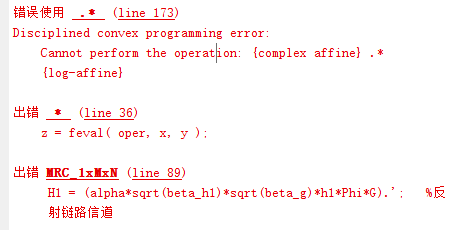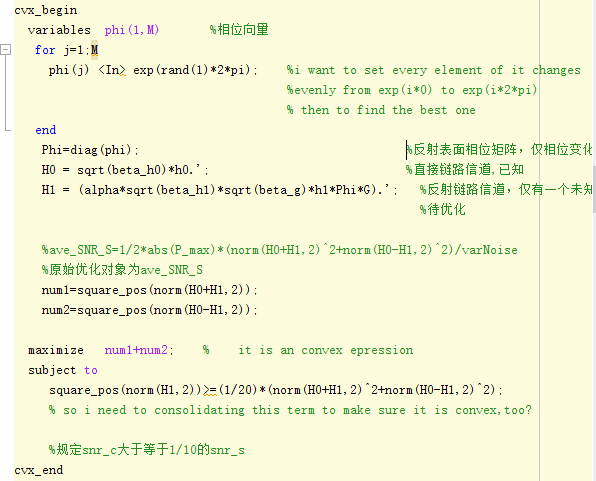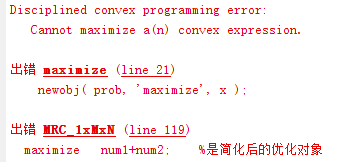# Disciplined convex programming error: Cannot perform the operation: {complex affine} .* {log-affine}when putting H1 outside of the cvx, ,it works fine.but when i put it into the cvx, it warns and can’t go on.

``````cvx_begin
variables  phi(1,M)             %相位向量
for j=1;M
phi(j) <In> rand(1)*2*pi;    %每个相位服从0-1随机均匀分布
a(j)=exp(i*phi(j));               %每个变量转换成服从0-2*Pi均匀分布
%a(j)=cos(phi(j))+sin(phi(j))*1i;
end
Phi=diag(a);                     %反射表面相位矩阵，仅相位变化
H0 = sqrt(beta_h0)*h0.';                            %直接链路信道
H1 = (alpha*sqrt(beta_h1)*sqrt(beta_g)*h1*Phi*G).';   %反射链路信道

%ave_SNR_S=1/2*abs(P_max)*(norm(H0+H1,2)^2+norm(H0-H1,2)^2)/varNoise
%原始优化对象为ave_SNR_S
num1=square_pos(norm(H0+H1,2));
num2=square_pos(norm(H0-H1,2));

minimize      1/(num1+num2);   %是简化后的优化对象
subject to
norm(H1,2)^2/varNoise>=(1/20)*(norm(H0+H1,2)^2+norm(H0-H1,2)^2);
%规定snr_c大于等于1/10的snr_s
cvx_end``````

only the phi is unknown and i want every element of it follows evenly distribution, so i put these limitation factor into the problem.please help me.

This is a horrible mess. You are volating many rules and much syntax. You should carefully re-read the CVX User’s Guide.

So let me start with this, how have you proven that this is a convex optimization problem?

i think the sum of two type 2 norm is still convex and for the variable phi ，it is a convex set。but now i’m not sure whether the relationship between the variable and the objective function is still convex。what if i set exp(j*phi) as the variable?will their relation become more simple? i really thanks for your guiding.

The sum of squared two-norms is convex (you never even get to that point, because the argument of norm is not affine). But you try to minimize the reciprocal of that, which is not convex.

And you have a two-norm squared on the left-hand side of `>=` constraint. That is non-convex. If the constraint really is convex, that will require consolidating the norm terms and being left on the RHS with a sum of convex terms.

There’s plenty more screwed up stuff with your program. There are some strange things in your program, for which I have no idea what the behavior of CVX is or “is supposed to” be. You define a variable, then an expression in terms of that variable (so far, that would be o.k,), but then you assign the variable in terms of the expression you assigned in terms of that variable (I don’t know how that works). I have no idea what `phi(j) <In> rand(1)*2*pi` does (apparently some kind of constraint is intended), but I doubt it does what you want.

Anyhow, I really didn’t want to get into all that, so I stated before, as I state again, that you need to show this problem is convex, which looks rather dubious to me, to put it mildly.